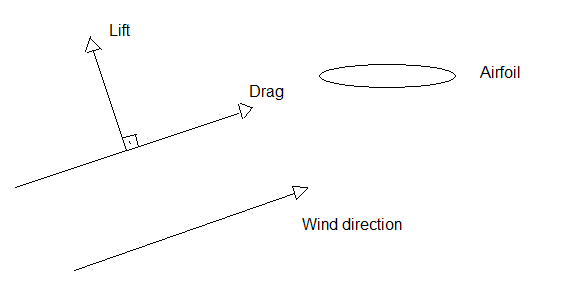# Drag Coefficient result?

#1

Hi , I am trying to find the drag coefficient for a body, however when I set up a result control I get one value, but when I go into para view and do calculations though there I get another value.

I get a much lower drag coefficient in para view, then in the result control, so my question is how is Simscale calculating the drag coefficient?

In ParaView I use this equation for drag coefficient : Cd = D / (A * .5 * r * V^2)
where D, drag is in newtons, A is reference in meters, r is density of fluid, and v is velocity of fluid.

Is there a way to export a simulation runs' cell data for the viscous forces vectors?
Post Processing Viscous Forces
#2

These resources might be helpful for you:

Also feel free to share your project with us so that we can see what setup you have chosen.

Cheers,

Jousef

Drag coefficient from wind on structure
#3

@bluematt2002 I presume you are neglecting viscous forces in your paraview calculation? The platform takes this into account for drag coefficient.

You can add the wall shear stress as a result control and use it to calculate the drag component due to viscous forces. If this still produces different results, can you share your method of calculation in paraview?

Best,
Darren

#4

yes I have wall shear stress as a result control, however I don’t know how to incorporate this into the equation.
My current set up is just calculating drag, then I find the drag coefficient by hand as that is a little faster.
in ParaView, to find drag I have: Normals_zp-1

#5

So for pressure forces you probably do surface normals times pressure. Then integrated respect to area will give forces in x, y and z. For viscous wall shear stress is just force/area so you can do wall shear integrated respect to area, giving the viscous forces in cuz, where the z components in your case should give the total drag force to use in your hand calculations.

Best,
Darren

#6

what do you mean by integrated? how do I integrate with the calculator filter?

Integrate Variables?

#7

Hi @bluematt2002, yes integrate variables.

Best,
Darren

#8

When I integrate the wallshearstress_z, is the result given in Pa?

#9

The theory is that tau=f/a integrated f/a.da=f therefore Newton’s.

Best,
Darren

#10

Hey, i have the same problem! My results of Lift and Drag are different on Paraview. What do you made to solve that? I don’t know which one i chose

#11

Hey @JPzanca,

Have a look at this thread. Best approach is to rotate the directions for Cd and Cl based on AoA (assuming you’re dealing with airfoils).

For reference (sorry for my bad paint skills)